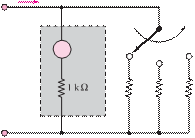### Create an Account

Already have account?

### Forgot Your Password ?

Home / Questions / Consider the practical ammeter, described in Figure P273 consisting of an ideal ammeter in...

# Consider the practical ammeter, described in Figure P273 consisting of an ideal ammeter in series with a 1kQ resistor The meter sees a full scale deflection when the current through it is

Consider the practical ammeter, described in Figure P2.73, consisting of an ideal ammeter in series with a 1-kresistor. The meter sees a full-scale deflection when the current through it is 30 μA. If we desire to construct a multirange ammeter reading

full-scale values of 10 mA, 100 mA, and 1 A, depending on the setting of a rotary switch, determine appropriate values of R1, R2,

and R3.I

Switch

A

VS          rm                   V

Source               Load Voltmeter

R= 25 kÙ

V12 V

Jun 19 2020 View more View Less

#### Answer (Solved)Subscribe To Get Solution